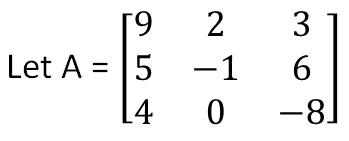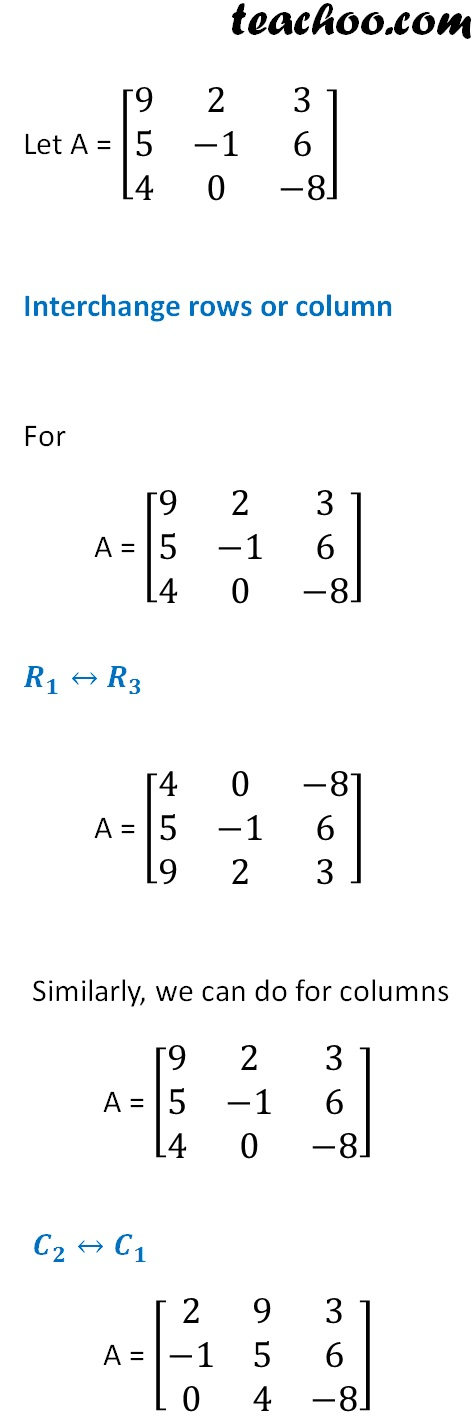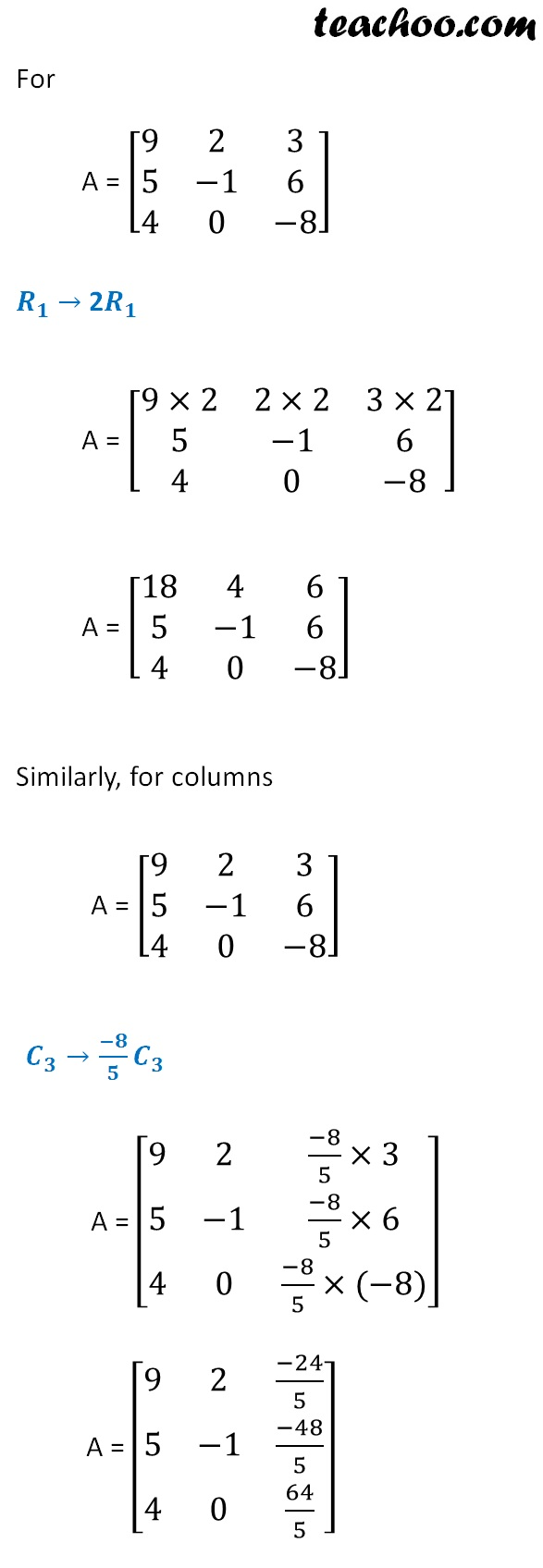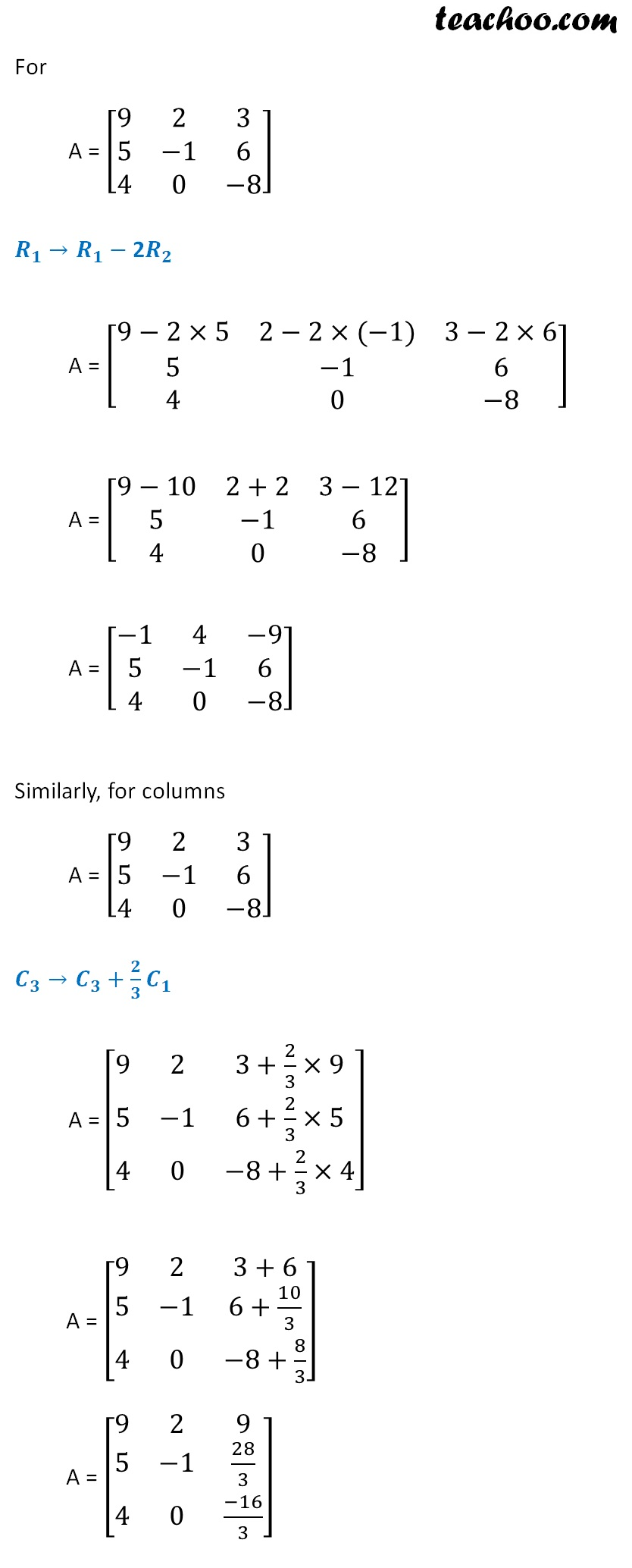Inverse of matrix using elementary transformation

Chapter 3 Class 12 Matrices
Concept wise

We use elementary operations to find inverse of a matrix

The elementary matrix operations are

• Interchange two rows, or columns
Example - R 1 ↔ R 3 , C 2 ↔ C 1

• Multiply a row or column by a non-zero number
Example - R 1 →2R 1 , C 3 →(-8)/5 C 3

• Add a row or column to another, multiplied by a non-zero
Example - R 1 → R 1 − 2R 2 ,  C 3 → C 3 + 2/3 C 1

Let’s look at each one of them in detail## Interchange rows or column## Multiply a row or column by a non-zero number## Add a row or column to another, multiplied by a non-zero numberLearn in your speed, with individual attention - Teachoo Maths 1-on-1 Class